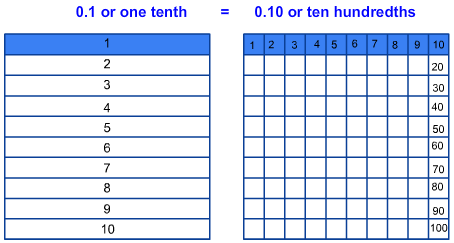# Equivalent Decimals

Print Rate 0 stars Common Core
Lesson size:
Message preview:
Someone you know has shared lesson with you:

To play this lesson, click on the link below:

https://www.turtlediary.com/lesson/equivalent-decimals.html

Hope you have a good experience with this site and recommend to your friends too.

Login to rate activities and track progress.
Login to rate activities and track progress.

Equivalent decimals are exactly what the name sounds like, decimals that are equal. For example, 0.1 (one tenth) is equal to 0.10 (ten hundredths).

Let's take a look at a model given below to understand equivalent decimals in a better way.From the model, it is clear that 0.1 is equal to 0.10. That is because, each tenth has been broken up into ten equal parts.

And if we break the hundredths into ten equal parts, then we will have 0.100 (one hundred thousandths), which also equals 0.1.

Below given are the two important concepts to understand equivalent decimals:

• It is important to know the difference between 0.1, 0.10 and 0.100. The place value indicates the parts of a whole get progressively smaller from tenths, hundredths to thousandths. That is because, as we move to the right of the decimal point, each position is 10 times less.
• The other important thing is that 0.10 and 0.100 can both be simplified to 0.1 The additional zeros to the right of 0.1 are called place holders and show the specific place value of decimal number.

Here are some other models that show equivalent decimals.Here are some more examples of equivalent decimals, without a model:

0.3 = 0.30 0.19 = 0.190 0.03 = 0.030 0.468 = 0.4680

In fact, any number of zeros (0) to the right of the last digit other than zero (1-9) is an equivalent decimal to the same decimal number without the zeros.

For example:

0.2 = 0.20
0.44 = 0.440
0.791 = 0.7910
0.2 = 0.200
0.44 = 0.4400
0.791 = 0.79100
0.2 = 0.2000
0.44 = 0.44000
0.791 = 0.791000
0.2 = 0.20000
0.44 = 0.440000
0.791 = 0.7910000

Let's take a look at some more examples.

## Example 1

Which of the following decimals is equivalent to 0.4?

a) 0.04

b) 0.14

c) 0.400

Any decimal number with additional zeros to the right of last digit other than zero (1-9) is equivalent to the decimal number without the zeros.

So, the correct answer is 0.400.

Example 2

Which of the following decimals is equivalent to 0.38?

a) 0.0038

b) 0.380

c) 3.8

Any decimal number with additional zeros to the right of last digit other than zero (1-9) is equivalent to the decimal number without the zeros.

So, the correct answer is 0.380.

## Example 3

Which of the following decimals is equivalent to 0.0480?

a) 4.80

b) 0.048

c) 0.00048

Look at the last digit to the right that is not a zero (any digit 1-9). That is the digit 8 in the thousandths place. Simplify by removing the zero farthest to the right of the decimal point after digit 8, like this: 0.0480

So, the correct answer is 0.048.

## Example 4

Which of the following decimals is equivalent to 0.31000?

a) 0.31

b) 0.0031

c) 3.10

Look at the last digit to the right that is not a zero (any digit 1-9). That is the digit 1.

Simplify by removing the 3 zeros farthest to the right of the decimal point after the digit 1, like this: 0.31000

So, the correct answer is 0.31.

## Equivalent Decimals

• Equivalent decimals are any decimal numbers that are equal.
• Decimal models show how equivalent decimals fill up the same amount of the whole.
• Any number of zeros (0) to the right of the last digit other than zero (1-9) is an equivalent decimal to the same decimal number without the zeros. For example: 0.2 = 0.20 = 0.200 = 0.2000.

## Similar Lessons

Become premium member to get unlimited access.